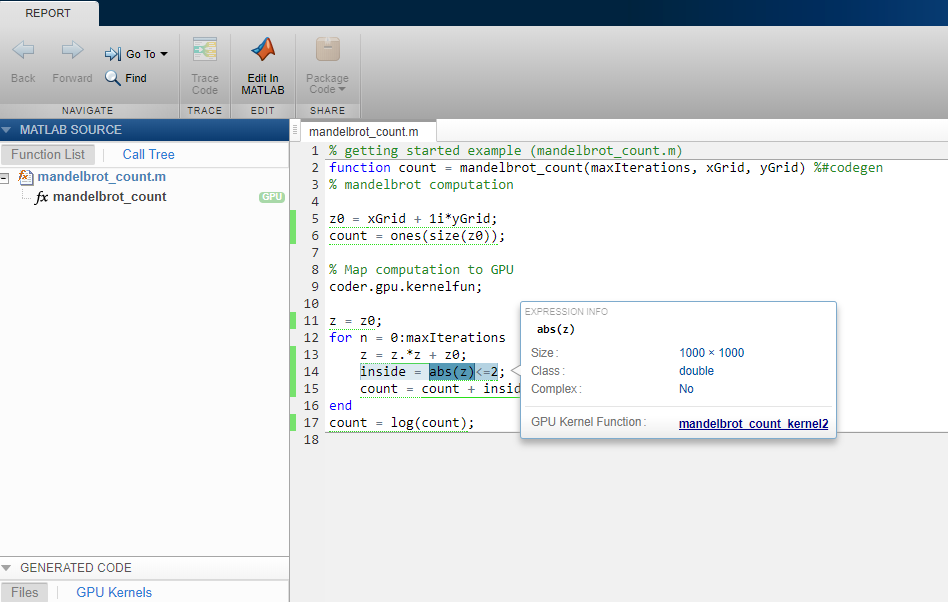## Trace Between Generated CUDA Code and MATLAB Source Code

This example shows how to trace (highlight sections) between MATLAB® source code and the generated CUDA® code. Tracing between source code and generated code helps you to:

• Understand how the code generator maps your algorithm to GPU kernels.

• Debug issues in the generated code.

• Evaluate the quality of the generated code.

You can trace by using one of these methods:

• Configure GPU Coder™ to generate code that includes the MATLAB source code as comments. In the comments, a traceability tag immediately precedes each line of source code. The traceability tag provides details about the location of the source code. If you have Embedded Coder®, in the code generation report, the traceability tags link to the corresponding MATLAB source code.

• With Embedded Coder, produce a code generation report that includes interactive traceability. Interactive tracing in the report helps you to visualize the mapping between the MATLAB source code and the generated C/C++ code. See Interactively Trace Between MATLAB Code and Generated C/C++ Code (Embedded Coder).

### Generate Traceability Tags

#### Create the MATLAB Source Code

To illustrate traceability tags, this example uses an implementation of the Mandelbrot set by using standard MATLAB commands running on the CPU. This implementation is based on the code provided in the Experiments with MATLAB e-book by Cleve Moler.

The Mandelbrot set is the region in the complex plane consisting of the values z0 for which the trajectories defined by this equation remain bounded at k→∞.

`${z}_{k+1}={z}_{k}^{2}+{z}_{0},\text{ }k=0,\text{\hspace{0.17em}}1,\text{ }\text{\hspace{0.17em}}\dots$`

Create a MATLAB function called `mandelbrot_count.m` with the following lines of code. This code is a vectorized MATLAB implementation of the Mandelbrot set. For every point `(xGrid,yGrid)` in the grid, it calculates the iteration index `count` at which the trajectory defined by the equation reaches a distance of `2` from the origin. It then returns the natural logarithm of `count`, which is used generate the color coded plot of the Mandelbrot set.

```function count = mandelbrot_count(maxIterations,xGrid,yGrid) % Add kernelfun pragma to trigger kernel creation coder.gpu.kernelfun; % mandelbrot computation z0 = xGrid + 1i*yGrid; count = ones(size(z0)); z = z0; for n = 0:maxIterations z = z.*z + z0; inside = abs(z)<=2; count = count + inside; end count = log(count); ```

#### Create Test Vectors

Create test vectors for the entry-point function by using the following lines of code. The script generates a 1000 x 1000 grid of real parts (x) and imaginary parts (y) between the limits specified by `xlim` and `ylim`. You can use these inputs to validate the `mandelbrot_count` entry-point function and plots the resulting Mandelbrot set.

```maxIterations = 500; gridSize = 1000; xlim = [-0.748766713922161,-0.748766707771757]; ylim = [0.123640844894862,0.123640851045266]; x = linspace(xlim(1),xlim(2),gridSize); y = linspace(ylim(1),ylim(2),gridSize); [xGrid,yGrid] = meshgrid(x,y); ```

#### Generate Traceability Tags

To produce traceability tags in the generated code, enable generation of MATLAB source code as comments.

• In the GPU Coder app, set MATLAB source code as comments to `Yes`.

• In a code generation configuration object, create a `coder.gpuConfig` object and set the `MATLABSourceComments` property to `true`.

```cfg = coder.gpuConfig('dll','ecoder',true); cfg.GenerateReport = true; cfg.MATLABSourceComments = true; cfg.GpuConfig.CompilerFlags = '--fmad=false'; codegen -config cfg -args {maxIterations,xGrid,yGrid} mandelbrot_count```

Note

The `--fmad=false` flag when passed to the `nvcc`, instructs the compiler to disable Floating-Point Multiply-Add (FMAD) optimization. This option is set to prevent numerical mismatch in the generated code because of architectural differences in the CPU and the GPU. For more information, see Numerical Differences Between CPU and GPU.

#### Access the Report

To open the code generation report, click View report.

The code generation report is named `report.mldatx`. It is located in the `html` subfolder of the code generation output folder. If you have MATLAB R2018a or later, you can open the `report.mldatx` file by double-clicking it.

In the MATLAB Source pane, select `mandelbrot_count.m`. You see the MATLAB source code in the code pane.The green GPU marker next to `mandelbrot_count` function indicates that the generated code has both CPU and GPU sections. The green vertical bar indicates the lines of code that are mapped to the GPU. To see information about the type of a variable or expression and the name of the corresponding GPU Kernel Function, pause over the variable or expression. When you select highlighted code by clicking it, the code becomes blue and you can see the information even when you move your pointer away from the selection. The code remains selected until you press `Esc` or select different code.

To view the CUDA code generated for the `mandelbrot_count.m` entry-point function, select `mandelbrot_count.cu` from the Generated Code pane.

### Format of Traceability Tags

In the generated code, traceability tags appear immediately before the MATLAB source code in the comment. The format of the tag is:
`<filename>:<line number>`.

For example, this comment indicates that the code ```z0 = xGrid + 1i*yGrid;``` appears at line `5` in the source file `mandelbrot_count.m`.

```/* 'mandelbrot_count:5' z0 = xGrid + 1i*yGrid; ```

### Traceability Tag Limitations

• You cannot include MATLAB source code as comments for:

• MathWorks® toolbox functions

• P-code

• The appearance or location of comments can vary:

• Even if the implementation code is eliminated, for example, due to constant folding, comments can still appear in the generated code.

• If a complete function or code block is eliminated, comments can be eliminated from the generated code.

• For certain optimizations, the comments can be separated from the generated code.

• Even if you do not choose to include source code comments in the generated code, the generated code includes legally required comments from the MATLAB source code.

• Functions with multiple outputs do not get highlighted.

• Calls to `coder` functions such as `coder.nullcopy` will not be highlighted

• Code that gets mapped to library calls such as cuDNN, cuBLAS and cuFFT will not be highlighted. As a result, functions that are completely mapped to GPU may be tagged incorrectly.